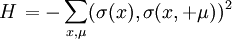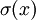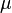# RP(n-1) modelThis article is a 'stub' page, it has no, or next to no, content. It is here at the moment to help form part of the structure of SklogWiki. If you add sufficient material to this article then please remove the {{Stub-general}} template from this page.$\left. H \right. = - \sum_{x, \mu} ( \sigma(x) , \sigma (x, + \mu))^2$
where H is the Hamiltonian of the system, the variables$\sigma (x)$ are n-component unit vectors of sites x of a square lattice, and$\mu$ denotes the two directions of the lattice. The n=2 case of the model is equivalent to the XY model.